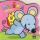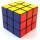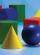# Granite cube

What is the weight in kg granite cube with an edge of 0.5 m if 1dm3 of granite weight 2600 g?

Result

m =  325 kg

#### Solution:Leave us a comment of this math problem and its solution (i.e. if it is still somewhat unclear...):Be the first to comment!#### Following knowledge from mathematics are needed to solve this word math problem:

Do you know the volume and unit volume, and want to convert volume units? Tip: Our Density units converter will help you with the conversion of density units. Do you want to convert mass units?

## Next similar math problems:

1. Transforming cuboidCuboid with dimensions 6 cm, 10, and 11 cm is converted into a cube with the same volume. What is its edge length?
2. HoleIn the center of the cube with edge 14 cm we will drill cylinder shape hole. Volume of the hole must be 27% of the cube. What drill diameter should be chosen?
3. LatheFrom the cube of edge 37 cm was lathed maximum cylinder. What percentage of the cube is left as waste after lathed?
4. Mouse HryzkaMouse Hryzka found 27 identical cubes of cheese. She first put in a large cube out of them and then waited for a while before the cheese cubes stuck together. Then from every wall of the big cube she will eats the middle cube. Then she also eats the cube
5. Volume from surface areaWhat is the volume of the cube whose surface area is 96 cm2?
6. CubeThe cube weighs 11 kg. How weight is cube of the same material, if its dimensions are 3-times smaller?
7. CubesCube, which consists of 27 small cubes with edge 4 dm has volume:
8. Cube in a sphereThe cube is inscribed in a sphere with volume 9067 cm3. Determine the length of the edges of a cube.Calculate the edge of the cube made ​​from lead, which weighs 19 kg. The density of lead is 11341 kg/m3.
10. TerezaThe cube has area of base 256 mm2. Calculate the edge length, volume and area of its surface.
11. Cube zoomHow many percents do we increase the volume and surface of the cube if we magnify its edge by 38 %?
12. Space diagonalThe space diagonal of a cube is 129.91 mm. Find the lateral area, surface area and the volume of the cube.
13. CubesOne cube is inscribed sphere and the other one described. Calculate difference of volumes of cubes, if the difference of surfaces in 257 mm2.
14. Cube in ballCube is inscribed into sphere of radius 241 cm. How many percent is the volume of cube of the volume of sphere?
15. CuboidFind the cuboid that has the same surface area as the volume.
16. Center of the cubeCenter of the cube has distance 33 cm from each vertex. Calculate the volume V and surface area S of the cube.
17. Cone in cubeThe cube is inscribed cone. Determine the ratio of the volume of cone and cube. The ratio express as a decimal number and as percentage.